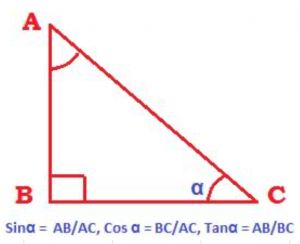## 1. Trig Identities on the six trigonometric ratios:

(Note: trig is an abbreviation for trigonometric. We will use trig for the word trigonometric to avoid repetition and therefore boredom.)1. Sinα = opposite side/hypotenuse = AB/AC
2. Cosα = adjacent side/hypotenuse = BC/AC
3. Tanα = opposite side/adjacent side = AB/BC

## 2. The reciprocal Trig identities

1. cosecα = 1/sinα
2. secα = 1/cosα
3. cotα = 1/tanα

## 3. The three Pythagorean trig identities

• Sin2α + Cos2α = 1
• Sec2α – tan2α = 1, Sec2α = 1+ tan2α
• Cosec2α – cotan2α = 1, Cosec2α = 1+ cotan2α

## 4. The quotient Trig identities:

• tan α = sin α /cos α
• cot α = cos α /sin α

## 5. Trig identities of negative angles:

• Sin (– α) = –sin α
• cos (– α) = cos α
• tan (– α) = – tanα
• cot (– α) = – cotα
• Sec (– α) = Sec α
• cosec (– α) = – cosec α

## 6. Trig identities of compound angles

• sin (A + B) = sinAcosB + cosAsinB
• sin (A – B) = sinAcosB – cosAsinB
• cos (A + B) = cosAcosB – sinAsinB
• cos (A – B) = cosAcosB + sinAsinB
• Tan(A + B) = (sinAcosB + cosAsinB)/(cosAcosB – sinAsinB)
• Tan(A – B) = (sinAcosB – cosAsinB)/(cosAcosB + sinAsinB)

## 7. Trig identities on products of compound angles

1. sin(A + B)sin(A – B) = sin2A – sin2B Or cos2B – cos2A
2. cos (A + B)cos(A – B) = cos2A – sin2B Or cos2B – sin2A
3. tan (A + B)tan(A – B) = (tan2A – tan2B)/ (1 – tan2Atan2B)
4. tan(A + B + C) = (tan A + tan B + tan C – tanA tanB tanC)/ (1– tanAtanB – tanB tanC –tanC tanA)

## 8. Trig identities on conversion of sum of angles to product of angles

• sin (A + B) + sin ( A – B) = 2sinAcosB
• sin (A + B) – sin (A – B) = 2 cosAsinB
• cos ( A + B ) + cos (A – B) = 2cosAcosB
• cos ( A – B ) – cos (A + B) = 2sinAsinB

## 10. trig identities on double angles:

• Sin2A = 2sinAcosA Or 2tanA/ (1 + tan2A)
• cos2A = 2cos2A – 1 Or 1 – 2sin2A Or (1 – tan2A)/(1 + tan2A)
• tan2A = 2tanA/(1 – tan2A)

## 11. Trig identities on triple angles

• Sin3A = 3sinA – 4sin3A
• cos3A = 4cos3A – 3cosA
• tan3A = (3tanA – tan3A)/(1 – 3tan2A)

## 12. Trig identities on sub-multiple angles

• sin2A + sin2B + sin2C = 4sinAsinBsinC Or -1 – 4cosAcosBcosC
• cos2A + cos2B + cos2C = 1 – 4 sinAsinBsinC
• tan2A + tan2B + tan2C = tan2C tan2B tan2C

## 14. the law of cosines or the cosine rule

• a2 = b2 + c2 – 2bc cosA
• b2 = a2 + c2 – 2ac cosB
• c2 = b2 + a2 – 2abcosC

## 15. the projection formula

• a = bcosC + ccosB
• b = acosC + ccosA
• c = bcosA + acosB